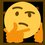# Explicit Formula for Fibonacci Sequence

Fibonacci Sequence: $1,1,2,3,5,8,13,21,\dots$

$\begin{cases}F_0=0\\F_1=1\\F_{n+2}=F_{n+1}+F_n\end{cases}$

$F_{n+2}-F_{n+1}-F_n=0$

Suppose $F_n=r^n$ solves this equation for some $r≠0$.

\begin{aligned} r^{n+2}-r^{n+1}-r^n&=0\\ r^n(r^2-r-1)&=0\\ r^2-r-1&=0\\ r&=\frac{1±\sqrt{5}}{2} \end{aligned}

$F_n=A\left(\frac{1+\sqrt{5}}{2}\right)^n+B\left(\frac{1-\sqrt{5}}{2}\right)^n$ solves $F_{n+2}-F_{n+1}-F_n=0$.

\begin{aligned} F_0&=A\left(\frac{1+\sqrt{5}}{2}\right)^0+B\left(\frac{1-\sqrt{5}}{2}\right)^0\\ 0&=A+B\\ B&=-A \end{aligned}

\begin{aligned} F_1&=A\left(\frac{1+\sqrt{5}}{2}\right)^1+B\left(\frac{1-\sqrt{5}}{2}\right)^1\\ 1&=A\left(\frac{1+\sqrt{5}}{2}\right)-A\left(\frac{1-\sqrt{5}}{2}\right)\\ 1&=A\left(\frac{1+\sqrt{5}}{2}-\frac{1-\sqrt{5}}{2}\right)\\ 1&=A\sqrt{5}\\ A&=\frac{1}{\sqrt{5}}\Rightarrow B=-\frac{1}{\sqrt{5}} \end{aligned}

\begin{aligned} F_n&=A\left(\frac{1+\sqrt{5}}{2}\right)^n+B\left(\frac{1-\sqrt{5}}{2}\right)^n\\ &=\frac{1}{\sqrt{5}}\frac{\left(1+\sqrt{5}\right)^n}{2^n}-\frac{1}{\sqrt{5}}\frac{\left(1-\sqrt{5}\right)^n}{2^n}\\ &=\frac{1}{\sqrt{5}}\left[\frac{\left(1+\sqrt{5}\right)^n}{2^n}-\frac{\left(1+\sqrt{5}\right)^n}{2^n}\right]\\ &=\frac{1}{\sqrt{5}}\frac{\left(1+\sqrt{5}\right)^n-\left(1-\sqrt{5}\right)^n}{2^n}\\ \end{aligned}

$\therefore\boxed{F_n=\frac{\left(1+\sqrt{5}\right)^n-\left(1-\sqrt{5}\right)^n}{2^n\sqrt{5}}}$

Proof by induction:

Base cases:

\begin{aligned} F_n\rvert_{n=0}&=\frac{\left(1+\sqrt{5}\right)^0-\left(1-\sqrt{5}\right)^0}{2^0\sqrt{5}}\\ &=\frac{1-1}{1\sqrt{5}}\\ &=\frac{0}{\sqrt{5}}\\ &=0\\ F_n\rvert_{n=0}&=F_0 \end{aligned}

\begin{aligned} F_n\rvert_{n=1}&=\frac{\left(1+\sqrt{5}\right)^1-\left(1-\sqrt{5}\right)^1}{2^1\sqrt{5}}\\ &=\frac{1+\sqrt{5}-1+\sqrt{5}}{2\sqrt{5}}\\ &=\frac{2\sqrt{5}}{2\sqrt{5}}\\ &=1\\ F_n\rvert_{n=1}&=F_1 \end{aligned}

Inductive hypothesis: assume $F_n=\frac{\left(1+\sqrt{5}\right)^n-\left(1-\sqrt{5}\right)^n}{2^n\sqrt{5}}$ is true for $n=k$.

$F_k=\frac{\left(1+\sqrt{5}\right)^k-\left(1-\sqrt{5}\right)^k}{2^k\sqrt{5}}$

\begin{aligned} F_{k+1}&=F_k+F_{k-1}\\ &=\frac{\left(1+\sqrt{5}\right)^k-\left(1-\sqrt{5}\right)^k}{2^k\sqrt{5}}+\frac{\left(1+\sqrt{5}\right)^{k-1}-\left(1-\sqrt{5}\right)^{k-1}}{2^{k-1}\sqrt{5}}\\ &=\frac{2\left(1+\sqrt{5}\right)^k-2\left(1-\sqrt{5}\right)^k+4\left(1+\sqrt{5}\right)^{k-1}-4\left(1-\sqrt{5}\right)^{k-1}}{2^{k+1}\sqrt{5}}\\ &=\frac{2\left(1+\sqrt{5}\right)^{k-1}\left(1+\sqrt{5}+2\right)-2\left(1-\sqrt{5}\right)^{k-1}\left(1-\sqrt{5}+2\right)}{2^{k+1}\sqrt{5}}\\ &=\frac{\left(1+\sqrt{5}\right)^{k-1}\left(2+2\sqrt{5}+4\right)-\left(1-\sqrt{5}\right)^{k-1}\left(2-2\sqrt{5}+4\right)}{2^{k+1}\sqrt{5}}\\ &=\frac{\left(1+\sqrt{5}\right)^{k-1}\left(1+2\sqrt{5}+5\right)-\left(1-\sqrt{5}\right)^{k-1}\left(1-2\sqrt{5}+5\right)}{2^{k+1}\sqrt{5}}\\ &=\frac{\left(1+\sqrt{5}\right)^{k-1}\left(1+\sqrt{5}\right)^2-\left(1-\sqrt{5}\right)^{k-1}\left(1-\sqrt{5}\right)^2}{2^{k+1}\sqrt{5}}\\ F_{k+1}&=\frac{\left(1+\sqrt{5}\right)^{k+1}-\left(1-\sqrt{5}\right)^{k+1}}{2^{k+1}\sqrt{5}}\quad\blacksquare \end{aligned}Note by Gandoff Tan
1 year, 8 months ago

This discussion board is a place to discuss our Daily Challenges and the math and science related to those challenges. Explanations are more than just a solution — they should explain the steps and thinking strategies that you used to obtain the solution. Comments should further the discussion of math and science.

When posting on Brilliant:

• Use the emojis to react to an explanation, whether you're congratulating a job well done , or just really confused .
• Ask specific questions about the challenge or the steps in somebody's explanation. Well-posed questions can add a lot to the discussion, but posting "I don't understand!" doesn't help anyone.
• Try to contribute something new to the discussion, whether it is an extension, generalization or other idea related to the challenge.

MarkdownAppears as
*italics* or _italics_ italics
**bold** or __bold__ bold
- bulleted- list
• bulleted
• list
1. numbered2. list
1. numbered
2. list
Note: you must add a full line of space before and after lists for them to show up correctly
paragraph 1paragraph 2

paragraph 1

paragraph 2

[example link](https://brilliant.org)example link
> This is a quote
This is a quote
    # I indented these lines
# 4 spaces, and now they show
# up as a code block.

print "hello world"
# I indented these lines
# 4 spaces, and now they show
# up as a code block.

print "hello world"
MathAppears as
Remember to wrap math in $$ ... $$ or $ ... $ to ensure proper formatting.
2 \times 3 $2 \times 3$
2^{34} $2^{34}$
a_{i-1} $a_{i-1}$
\frac{2}{3} $\frac{2}{3}$
\sqrt{2} $\sqrt{2}$
\sum_{i=1}^3 $\sum_{i=1}^3$
\sin \theta $\sin \theta$
\boxed{123} $\boxed{123}$

Sort by:

Wow!!!!!!!!!!!!!!!!!!! @Gandoff Tan

- 1 year, 2 months ago

The proof has used techniques to solve a recursion. Nice!

- 1 year, 2 months ago

What does n|n=1 mean? In Hungary we use a|b if a%b=0.

- 1 year, 1 month ago